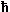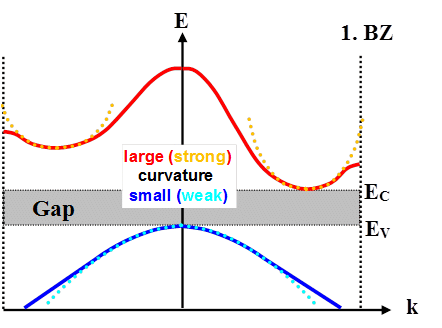2.3.1 Effective MassesSo far we have treated electrons and holes as identical (except for their charge and density) and we also always assumed thermal equilibrium.On occasion, we were somewhat inconsistent about the issues. We stated that p-n junctions with external voltages are actually not in equilibrium, or we kept the electron and hole parts of the currents separate, but we did not really make much of this – in such situation, we used equilibrium formulas, or did not justify why we do not mix electron and hole currents in the junction.In this subchapter we delve deeper into some "advanced" subjects (they are still pretty elementary) to get at least these aspects straight.First, we will see how we can save large parts of the simplicity of the free electron gas model by assigning effective masses to the carriers.In the free electron gas model an electron had the mass me (always written straight and not in italics because it is not a variable but a constant of nature (disregarding relativistic effects for the moment), and from now on without the subscript e) – and that was all to it.If a force F acts on it, e.g. via an electrical field E, in classical mechanics Newtons laws applies and we can write
F  = – e · E  =  m · d2r
dt2With r = position vector of the electron.An equally valid description is possible using the momentum p which gives us
p  =  m · dr
dt

F  = –e · E  =   dp
dtQuantum mechanics might be different from classical mechanics, so let's see what we get in this case.The essential relation to use is the identity of the particle velocity with the the group velocity vgroup of the wave package that describes the particle in quantum mechanics. The equation that goes with it is
vgroup  =  1·  Ñk E(k)Let's see what we get for the free electron gas model. We had the following expression for the energy of a quasi-free particle:
E(k)  =  Ekin   =2 · k2
2mFor vgroup we then obtain
vgroup  =  1·k2 · k2
2m
=· k
m
=  p
m
=  vclassicSincek was equal to the momentum p = mvclassic of the particle, we have indeed vgroup = vclassic = vphase as it should be.In other words: As long as the E(k) curve is a parabola, all the energy may be interpreted as kinetic energy for a particle with a (constant) mass m.Contrariwise, if the dispersion curve is not a parabola, not all the energy is kinetic energy (or the mass is not constant?).How does this apply to an electron in a periodic potential?We still have the wave vector k, butk is no longer identical to the momentum of an electron (or hole), but is considered to be a crystal momentum.E(k) is no longer a parabola, but a more complicated function.Since we usually do not know the exact E(k) relation, we seem to be stuck. However, there are some points that we still can make:Electrons at (or close to) the Brillouin zone edge in each band are diffracted and form standing waves, i.e. they are described by superpositions of waves with wave vector k and k. Their group velocity is necessarily close to zero!This implies that ÑkE(k) at the BZ edge must be close to zero too, which demands that the dispersion curve is almost horizontal at this point.The most important point is: We are not interested in electrons (or holes) far away from the band edges. Those electrons are just "sitting there" (in k-space) and not doing much of interest; only electrons and holes at the band edges (characterized by a wave vector kex) participate in the generation and recombination processes that are the hallmark of semiconductors.We are therefore only interested in the properties of these electrons and holes – and consequently only that part of the dispersion curve that defines the maxima or minima of the valence band or conductance band, respectively, is important.The thing to do then is to expand the E(k) curve around the points kex of the extrema into a Taylor series, written, for simplicities sake, as a scalar equation and with the terms after k2 neglected.
 En(k)  ¶En =  EV,C  + k  · ¶k ÷ ÷ ÷ ÷Since we chose the extrema of the dispersion curve, we necessarily have
¶En ¶k ÷ ÷i.e. we are looking at the top of the valence and the bottom of the conductance band.This leaves us with
En(k)  =   EV,C  + ÷ ÷If we now look at the conduction band and consider only the deviation from its bottom (the zero point of the energy scale usually is at the top of the valence band), we have the same quadratic relation in k as for the free electron gas, provided we change the definition of the mass as follows:
¶2EC ¶k2 ÷ ÷which permits to rewrite the Taylor expansion for the conduction band as follows
EC(k)  –  Eg2
2m*
· k2And this is the same form as the dispersion relation for the free electron gas!However, since 2EV,C/k2 may have arbitrary values, the effective mass m* of the quasi-free particles will, in general, be different from the regular electron rest mass m.We therefore used the symbol m* which we call the effective mass of the carrier and write it in italics, because it is no longer a constant but a variable. It is defined by

m*  =2 ·   1
2En/k2 |kexThe decisive factor for the effective mass is thus the curvature of the dispersion curve at the extrema, as expressed in the second derivative. Large curvatures (= large second derivative = small radius of curvature) give small effective masses, small curvatures (= small second derivative = large radius of curvature) give large ones.Let's look at what we did in a simple illustration and then discuss what it all means.Shown is a band diagram not unlike Si. The true dispersion curve has been approximated in the extrema by the parabola resulting from the Taylor expansion (dotted lines). The red ones have a larger curvature (i.e. the radius of an inscribed circle is small); we thus expect the effective mass of the electrons to be smaller than the effective mass of the holes.The effective mass has nothing to do with a real mass; it is a mathematical contraption. However, if we know the dispersion curves (either from involved calculations or from measurements), we can put a number to the effective masses and find that they are not too different from the real masses.This gives a bit of confidence to the following interpretation (which can be fully justified theoretically):If we use the effective mass m* of electrons and holes instead of their real mass m, we may consider their behavior to be identical to that of electrons (or holes) in the free electron gas model.This applies in particular to their response to forces. In this case, the deviation from the real mass takes care of the influence of the lattice on the movement of the particle.Taken to the extremes, this may even imply zero or negative effective masses. (Yes, even zero effective mass is possible, but this is a special case of its own and will not be discussed here; for example, graphene has some k-points where m* = 0.)As one can see from the dispersion curves, the effective mass of the electrons in the valence band, m*V, is always negative. (This means that a force in +x direction will cause such an electron to move in x direction.)Since this is counter-intuitive, usually one doesn't consider the electrons in the valence band, but just the unoccupied places – i.e., the holes; however, in their proper definition (which we have disregarded so far), holes have a positive mass by setting
m*h := –m*VNote (again) that "holes" only exist in the valence band; this is due to their proper definition as quasi-particles contributing to the electrical conductivity. Thus, all other empty states – in the conduction band, or on additional levels (e.g. of dopant atoms) – are not holes; this is clear since they do not contribute to the electrical conductivity.We will not go into more detail but give some (experimental) values for effective masses:
 Holes: m*/m IV; IV-IV III-V II-VI IV-VI C 0.25 AlSb 0.98 CdS 0.80 PbS 0.25 Si 0.16 (0.49) GaN 0.60 CdSe 0.45 PbTe 0.20 Ge 0.04 (0.28) GaSb 0.40 ZnO ? SiC (a) 1.00 GaAs 0.082 ZnS ? GaP 0.60 InSb 0.40 InAs 0.40 InP 0.64
 Electrons: m*/m IV; IV-IV III-V II-VI IV-VI C 0.2 AlSb 0.12 CdS 0.21 PbS 0.25 Si 0.98 (0.19) GaN 0.19 CdSe 0.13 PbTe 0.17 Ge 1.64 (0.082) GaSb 0.042 ZnO 0.27 SiC (a) 0.6 GaAs 0.067 ZnS 0.40 GaP 0.82 InSb 0.014 InAs 0.023 InP 0.077If there are two values it simply means that two dispersion curves (from different branches in k-space) have an extremum at the same point.We already get a feeling that it may make a difference if you work with electrons or holes in a certain device whenever you consider its frequency limit: As soon as the carriers can no longer follow rapidly changing forces (alternating electrical fields at high frequencies), the device will not work anymore.Small effective masses mean small (apparent) inertia or high mobilities µ. Looking at one of the many formulas for mobility, µ = e · ts/ m, the mobility goes up if we insert the (smaller) effective mass. It may thus be wise to use p-doped Si if high frequencies matter (and everything else does not matter).We also notice that Ge has the smallest effective mass for holes!This means that its holes respond more quickly to the accelarating force of an electrical field and that means that they also can change direction more quickly than holes in other semiconductors if the electrical field changes its direction.In other words: If speed only depends on the effective mass of the carriers, Ge still "works" at high frequencies when the other semiconductors have given up!And that is why we now (2004) see a sudden revival of Ge, which after its brief period of glory in the end of the fifties / beginning of the sixties of the 20th century, when it was the one and only semiconductor used for single transistors, was all but extinct.

© H. Föll (Semiconductors - Script)